# Guide: Standard Deviation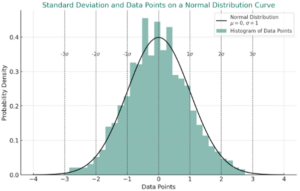The standard deviation is a crucial statistical measure in data analysis, especially within Lean Six Sigma methodologies. It quantifies the extent of variation or dispersion in a dataset, thereby illuminating how data points scatter around the mean. This understanding is pivotal not only for interpreting the data but also for assessing risks and making informed decisions.

In Lean Six Sigma, the standard deviation becomes a powerful tool, assisting in identifying process inefficiencies and quality inconsistencies and ultimately guiding improvements towards optimal operational efficiency.

## What is Standard Deviation?

The standard deviation is a statistical measure that can be used to understand the amount of variation or dispersion within a dataset. This measure helps us to understand how the individual data points in a data set are distributed around the mean (average value).

Understanding standard deviation as a measure is important for interpreting data, assessing risk, and making informed decisions and improvements within Lean Six Sigma.

### Standard Deviation in Lean Six Sigma

The standard deviation is a very commonly used tool in Lean Six Sigma for example:

A manufacturing company seeks to improve the efficiency and quality of its product assembly line. They record the time taken to assemble each unit over a month. Analyzing this data, they calculate the standard deviation to understand the variability in assembly times. A high standard deviation indicates significant inconsistencies in the production process, highlighting opportunities for process standardization and waste reduction.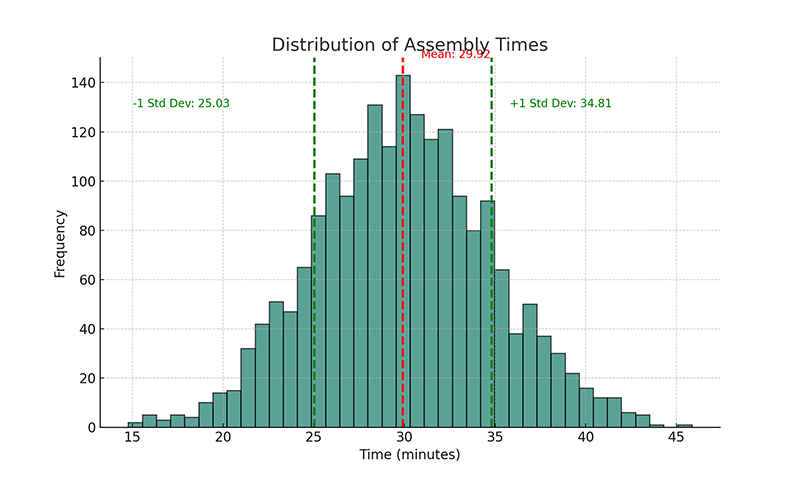From this, we can see The mean (average) assembly time is 29.92 minutes, and there is variation around that mean with a range from around 15 minutes to 45 minutes.

It could be possible that the assembly closer to 15 minutes is of poorer quality than the mean, and the assembly times around 45 minutes are inefficient methods.

By targeting these variations and implementing Lean Six Sigma methodologies to reduce the standard deviation, the company aims to achieve a more efficient, consistent production line, leading to higher-quality products and reduced operational costs.

## Understanding Standard Deviation

Standard deviation is a statistical measure that quantifies the amount of variation or dispersion in a set of data values. Therefore, A low standard deviation indicates that the values tend to be close to the mean (also called the expected value) of the set, while a high standard deviation indicates that the values are spread out over a wider range.

To illustrate this concept graphically, we can use a normal distribution curve, which is a common way of representing data in statistics. The normal distribution, also known as the bell curve, is symmetric and describes how the values of a dataset are distributed. Most of the observations cluster around the central peak, and the probabilities for values further away from the mean taper off equally in both directions.

### Visualizing Standard Deviation on a Normal Distribution Curve

1. Mean (Average): This is the central peak of the bell curve where the majority of values lie.
2. Standard Deviation: It measures the spread of data points. In a normal distribution:
• About 68% of values lie within one standard deviation of the mean.
• Approximately 95% are within two standard deviations.
• Around 99.7% fall within three standard deviations.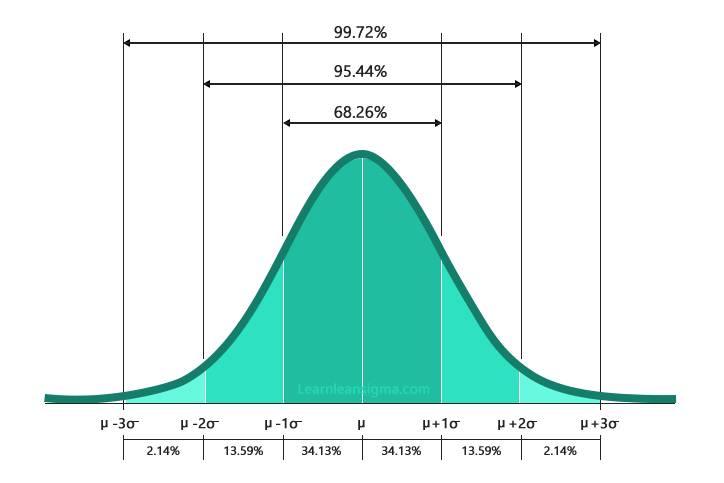### Example

For example the two graphs of normal distributions below. Both have the same mean, but different standard deviations to show how it affects the spread of data.

• Graph 1: Has a smaller standard deviation, representing data that is more clustered around the mean.
• Graph 2: Has a larger standard deviation, indicating more spread-out data.The two graphs above visually represent how standard deviation affects the spread of data in a normal distribution.

1. Graph 1 (Left): Normal Distribution with Smaller Standard Deviation

• Here, the curve is steeper and narrower. This indicates that most data points are clustered closely around the mean. There’s less variability, and therefore, a smaller standard deviation.
• In practical terms, if this were a set of test scores, for example, it would suggest that most students scored close to the average score, with few outliers.
2. Graph 2 (Right): Normal Distribution with Larger Standard Deviation

• This curve is flatter and wider, indicating that data points are spread out over a wider range. There’s more variability, hence a larger standard deviation.
• In the context of test scores, this would suggest a wide range of scores, from very low to very high, with students’ performances varying significantly.

## Options of Calculating Standard Deviation

### Quick Calculation Option

If you’re in a hurry or prefer a hassle-free approach, you can quickly calculate the standard deviation using our Standard Deviation Calculator. This tool is designed to make your life easier, especially when dealing with business metrics.

### Why Calculate Manually?

Understanding how to calculate the standard deviation manually provides you with a deeper grasp of the concept, which is essential for data-driven decision-making in business or Lean Six Sigma projects.

### The Formula

The formula for calculating the sample standard deviation () is: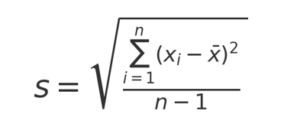## Step-by-Step Process to Calculate Standard Deviation

### Step 1: Calculate the Mean (Average)

The first step of the

The standard deviation calculation is to calculate the mean average value of the data set. This will be the central reference point for measuring deviations. So to calculate the mean, you add all the numbers in the data set together and divide by the number of data points.

For example: (10,15,12,8,11,14,13,9,10,16) / 10  = 11.8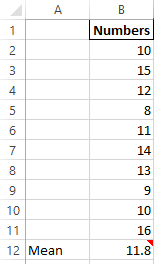### Step 2: Determine Each Data Point’s Deviation from the Mean

The next step is determining how far each point is from the mean. To find this, you subtract the mean from each data point.

For example, 10 is 1.8 less than the mean; therefore, the deviation would be -1.8.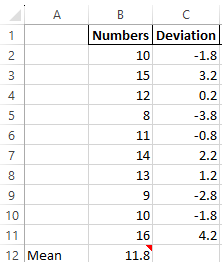### Step 3: Square Each Deviation

Next, we need to square each deviation. Squaring each deviation has two purposes:

1. It eliminates the negative value, which is important as we are interested in the amount of deviation and not the direction.
2. It gives more weight to larger deviations.

To square a number, you multiply the number by itself; for example, the first deviation squared will be 1.8 X 1.8 = 3.24. You can ignore the minus in front of the numbers for this.### Step 4: Find the Average of these Squares (Variance)

The next step is to find the average of the squares (variance). The variance measures how spread out the data is. To calculate variance, add all the squared deviations and divide by the number of data points.

In our example, the variance is (3.24 + 10.24 + 0.04 + 14.44 + 0.64 + 4.84 + 1.44 + 7.84 + 3.24 + 17.64 ) / 10 = 6.36### Step 5: Take the Square Root of the Variance (Standard Deviation)

Finally, to calculate the standard deviation, you need to calculate the square root of the variance. This step brings the units back to their original scale, which makes interpretation easier. For our data set, the standard deviation would be √6.36 ≈ 1.72.

The square root icon on your calculator will look like this:### Population vs. Sample Standard Deviation

There’s an important distinction between the standard deviation of a population and a sample:

• Population Standard Deviation is used when your data set includes the entire population you’re studying. The variance formula divides by ‘N’, the number of data points.
• Sample Standard Deviation is used when your data set is a sample representing a larger population. To account for the fact that you’re working with a sample, the variance formula divides by ‘N-1’ instead of ‘N’. This adjustment, known as Bessel’s correction, provides a more unbiased estimate of the population variance.

## Standard Deviation in Lean Six Sigma

In the world of Lean Six Sigma, standard deviation is more than just a statistical concept — it’s a cornerstone for improving processes and enhancing quality. Specifically, it plays a pivotal role in the Measure and Analyze phases of the DMAIC (Define, Measure, Analyze, Improve, Control) framework. Let’s delve into how standard deviation is applied in each of these contexts.

### Measure Phase: Identifying Process Variations

In the Measure phase, the primary objective is to quantify the current performance of the process you’re looking to improve. Standard deviation comes into play as a measure of process variability. For example, if you’re measuring the time it takes to assemble a product, a high standard deviation would indicate significant variations in assembly time. This would be an area to focus on for improvement, as variability often leads to inefficiencies and increased costs.

### Analyze Phase: Assessing the Stability of Processes

Once you’ve measured the key process metrics, the next step is to analyze them to identify root causes of problems or inefficiencies. A common tool used for this is the control chart, which plots process data over time. The standard deviation helps in assessing the ‘control limits’ of the chart. If data points fall outside of these limits (usually set at +/- 3 standard deviations from the mean), the process is considered unstable and requires further investigation.

### Setting Up Control Limits in Control Charts

Control limits in Lean Six Sigma are statistical boundaries that define the acceptable variation in a process. They are typically set at +/- 3 standard deviations from the process mean. When data points fall within these limits, the process is considered to be “in control,” meaning it’s stable and predictable. On the other hand, data points that fall outside these limits indicate that the process is “out of control,” suggesting that there are special causes of variation that need to be identified and addressed.

### Why Standard Deviation is Crucial in Lean Six Sigma

In summary, standard deviation serves as a quantifiable metric that allows Lean Six Sigma practitioners to:

1. Identify Variability: Understand how much fluctuation exists in a process.
2. Assess Stability: Determine whether a process is stable and predictable.
3. Set Control Limits: Establish boundaries for acceptable variation in a process.

Understanding and applying standard deviation in these contexts can significantly enhance your Lean Six Sigma projects, leading to more effective process improvements and, ultimately, higher quality outputs.

## Conclusion

In conclusion, standard deviation stands as a cornerstone in Lean Six Sigma, playing a vital role in both the Measure and Analyze phases of the DMAIC framework. It enables practitioners to quantify process variability, assess stability, and set accurate control limits, crucial for identifying and addressing inefficiencies.

By mastering standard deviation, you gain a deeper understanding of process behaviours, paving the way for significant improvements in quality and efficiency. Understanding and applying this concept effectively can dramatically enhance the outcomes of Lean Six Sigma projects, leading to more streamlined, predictable, and high-quality processes.

## References

A: Population standard deviation is used when you have data from every member of the population, whereas sample standard deviation is used when you only have a subset of the population’s data. The formula for sample standard deviation divides by  instead of to provide a more unbiased estimator.

A: No, standard deviation cannot be negative. It’s a measure of dispersion, and it wouldn’t make sense to have a negative distance from the mean. The square root in the standard deviation formula ensures that the result is non-negative.

A: Variance is the square of the standard deviation. While standard deviation gives you a measure of dispersion in the original units of the data, variance gives it in squared units. To convert variance to standard deviation, take the square root; to convert standard deviation to variance, square it.

A: In Lean Six Sigma, standard deviation is crucial for understanding process variability. It is used in the Measure and Analyze phases of the DMAIC framework to assess process stability, set control limits, and identify areas for improvement. A lower standard deviation often indicates a more stable process.

A: Yes, but with caution. Standard deviation is most informative for normal distributions. For skewed or bimodal distributions, other measures like interquartile range might be more appropriate. However, standard deviation can still provide a rough idea of data spread in non-normal distributions.

## Author#### Daniel Croft

Daniel Croft is a seasoned continuous improvement manager with a Black Belt in Lean Six Sigma. With over 10 years of real-world application experience across diverse sectors, Daniel has a passion for optimizing processes and fostering a culture of efficiency. He's not just a practitioner but also an avid learner, constantly seeking to expand his knowledge. Outside of his professional life, Daniel has a keen Investing, statistics and knowledge-sharing, which led him to create the website learnleansigma.com, a platform dedicated to Lean Six Sigma and process improvement insights.

## Free Lean Six Sigma Templates

Improve your Lean Six Sigma projects with our free templates. They're designed to make implementation and management easier, helping you achieve better results.